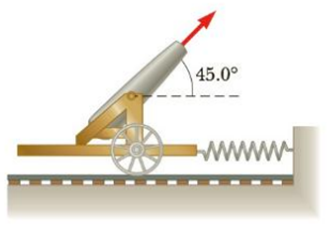Chapter 6, Problem 75AP

Chapter
Section
Textbook Problem

A cannon is rigidly attached to a carriage, which can move along horizontal rails, but is connected to a post by a large spring, initially unstretched and with force constant k = 2.00 × 104 N/m, as in Figure P6.75. The cannon fires a 2.00 × 102-kg projectile at a velocity of 125 m/s directed 45.0° above the horizontal. (a) If the mass of the cannon and its carriage is 5.00 × 103-kg, find the recoil speed of the cannon, (b) Determine the maximum extension of the spring, (c) Find the maximum force the spring exerts on the carriage, (d) Consider the system consisting of the cannon, the carriage, and the shell. Is the momentum of this system conserved during the firing? Why or why not?Figure P6.75

(a)

To determine
The recoil speed of the canon.

Explanation

Given info: The force constant if the spring connected to the canon is 2.00×104Nm-1 . The mass of the projectile fired is 2.00×102kg . The velocity of the projectile is 125ms-1 and directed at 45° above the horizontal. The mass of the carriage and canon is 5.00×103kg .

Explanation:

Linear momentum is conserved in both horizontal and vertical direction. From conservation of momentum along the horizontal direction,

(px)final=(px)initial

• (px)final is the total final momentum in the horizontal direction
• (px)final is the total initial momentum in the horizontal direction

The initial momentum of the system is zero, so the above expression becomes, (px)final=0

The total final momentum will be the sum of momentum of the projectile and the canon-carriage system

(b)

To determine
The maximum extension of the spring.

(c)

To determine
The maximum force the spring exerts on the carriage.

(d)

To determine
Whether the momentum of the system of canon, carriage and the shell is conserved.

Still sussing out bartleby?

Check out a sample textbook solution.

See a sample solution

The Solution to Your Study Problems

Bartleby provides explanations to thousands of textbook problems written by our experts, many with advanced degrees!

Get Started

Partially completed proteins are not held for completion at a later time when the diet may improve. T F

Nutrition: Concepts and Controversies - Standalone book (MindTap Course List)

If two stars differ by 8.6 magnitudes, what is their flux ration?

Horizons: Exploring the Universe (MindTap Course List)

2. In healthy men, the hematocrit is about

Cardiopulmonary Anatomy & Physiology

What is a chemical reaction?

Biology: The Dynamic Science (MindTap Course List)